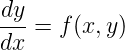﻿FunctionMath.IntegrateOde Method (Func(Double, Double, Double), Double, Double, Double, OdeSettings)Solves an ordinary differential equation initial value problem.

Namespace:  Meta.Numerics.Analysis
Assembly:  Meta.Numerics (in Meta.Numerics.dll) Version: 4.1.4Syntax
```public static OdeResult IntegrateOde(
Func<double, double, double> rhs,
double x0,
double y0,
double x1,
OdeSettings settings
)```

#### Parameters

rhs
Type: SystemFuncDouble, Double, Double
The right hand side function, which returns the value of the derivative given the values of the independent variable and the function.
x0
Type: SystemDouble
The initial value of the independent variable.
y0
Type: SystemDouble
The initial value of the function.
x1
Type: SystemDouble
The final value of the independent variable.
settings
Type: Meta.Numerics.AnalysisOdeSettings
The settings to use when solving the problem.

#### Return Value

Type: OdeResult
The solution, including the final value of the function and its derivative.Exceptions
ExceptionCondition
ArgumentNullExceptionThe rhs or settings is null.
NonconvergenceExceptionThe ODE could not be integrated to the required precision before exhausting the maximum allowed number of rhs evaluations.Remarks

An ordinary differential equation (ODE) has the form:The function specifying the derivative as a function of x and y is called the right-hand-side (RHS).

The integration of an ODE consists of specifying the value of y at some initial x and computing its value at a different x in accordance with the differential equation. The terms "initial" and "final" are derived from the common case where the independent variable is time, but the algorithm works whether the independent variable resents a time, a location, or a completely non-physical quantity, as long as the problem has the form of an ODE.

ODEs involving multiple, coupled dependent variables can be integrated using the IntegrateOde(FuncDouble, IReadOnlyListDouble, IReadOnlyListDouble, Double, IReadOnlyListDouble, Double, MultiOdeSettings) method. Higher order ODEs can be integrated by representing them as coupled ODEs in which the zeroth component is the desired y, the first component is y', the second component is y'', etc. So-called conservative second order ODEs should be integrated using the IntegrateConservativeOde(FuncDouble, Double, Double, Double, Double, Double, Double, OdeSettings) method. If your ODE's RHS depends only on x, the problem reduces to a simple integral, which can be solved more rapidly and accurately using the Integrate(FuncDouble, Double, Interval, IntegrationSettings) method. Analytic techniques can also be used to reduce several other kinds of ODEs to simple integrals or lower-order ODEs.See Also